Get instant live expert help with Excel or Google Sheets“My Excelchat expert helped me in less than 20 minutes, saving me what would have been 5 hours of work!”

#### Post your problem and you’ll get expert help in seconds.

Your message must be at least 40 characters
Our professional experts are available now. Your privacy is guaranteed.

# How to Extract a Substring in Excel – Excelchat

The Excel substring is part of the text string that we need to extract as a piece of information. We can extract the substring by manipulating the text string from a cell by using different methods in Excel. We need to learn how to extract a substring using Excel Text functions and other features.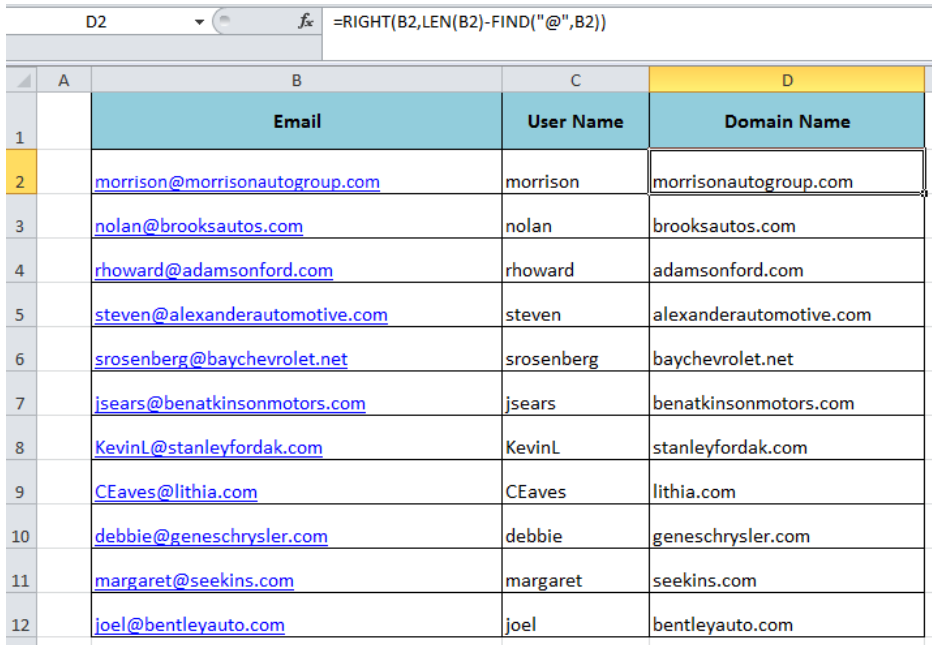Figure 1. How to Extract Substring in Excel

## Extract Substring Using Excel Text Functions

There are various Text functions like LEFT, RIGHT, MID which we use to extract substring in Excel from a text string. These functions are also knowns as substring functions but there is no specific Excel Substring function. There are other functions also, like FIND, SEARCH, LEN which we are used for identifying certain symbols and words to extract substring easily, like user name and the domain name from email address.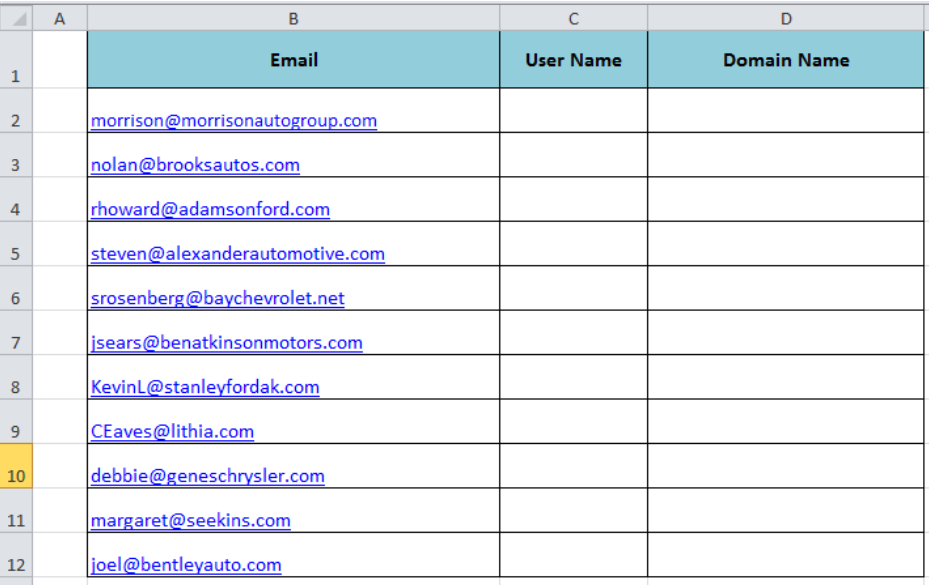Figure 2. Extracting Substring in Excel

### Using Excel LEFT Function

Excel LEFT function returns the specified number of character from the left side of the text string. We supply either a specified number of characters in the num_char argument or use Excel FIND or SEARCH function to return the position of any special character (as number) in the string to supply in the num_char argument. In this example, we need to extract User Name from email address as substring in the following formula;

`=LEFT(B2,FIND("@",B2)-1)`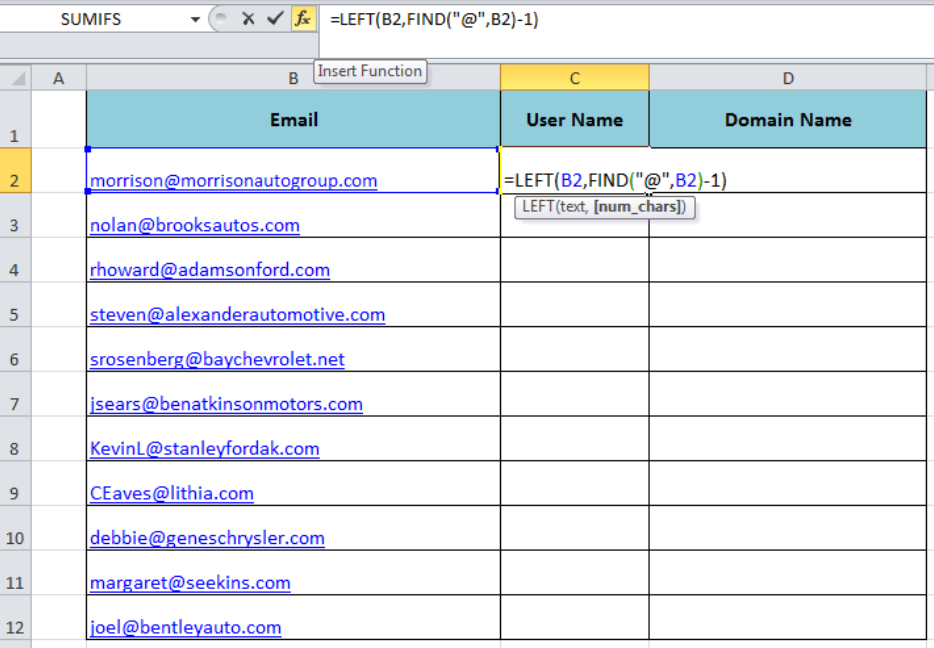Figure 3. Using Excel LEFT function

The Excel FIND function returns the position of special character “@” as a numeric value and 1 is subtracted from this numeric value to return the exact number of specific characters to extract the substringl from the left of the text string.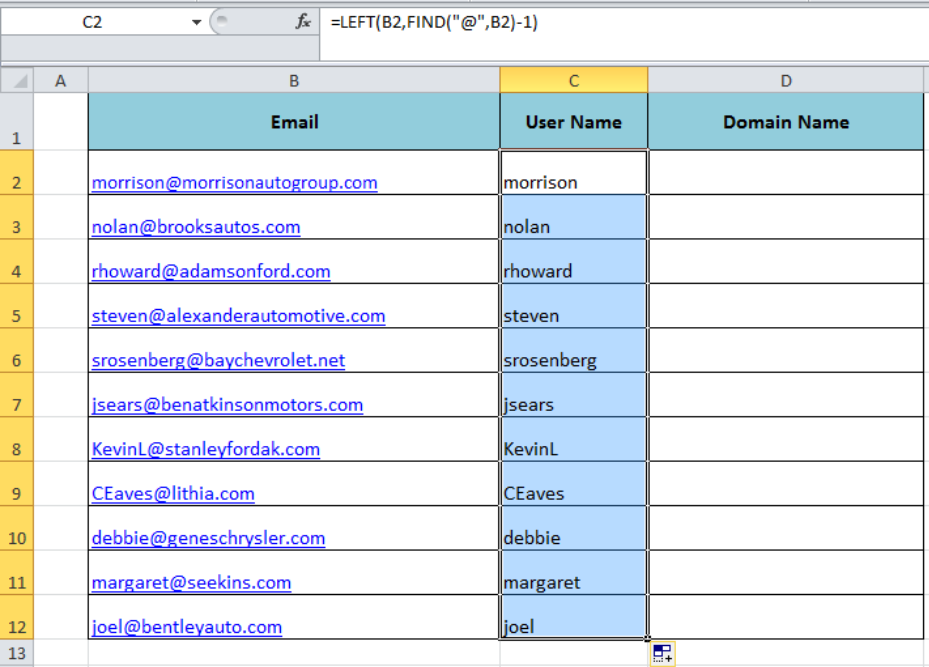Figure 4. Extracting Substring in Excel

### Using Excel RIGHT Function

The Excel RIGHT function returns the specified number of characters from the right of the text string by supplying the number of character in the num_char argument. If the pattern of the string is not the same then we use Excel LEN and FIND functions to return the specified number of characters. In our example, we extract Domain Name as Excel Substring from the right side of the email address in the following formula;

`=RIGHT(B2,LEN(B2)-FIND("@",B2))`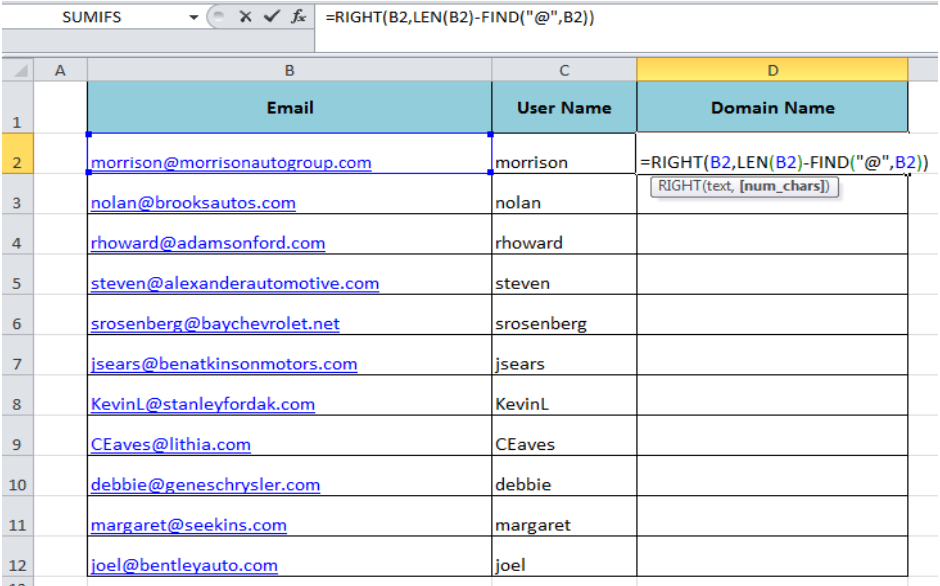Figure 5. Using Excel RIGHT Function

We use Excel LEN and FIND functions together in the num_char argument to return the specified number of characters. Excel FIND function returns the position of the symbol “@” and we subtract this number from the total length of the string returned by Excel LEN function to calculate the specified number of characters to extract the Domain Name substring.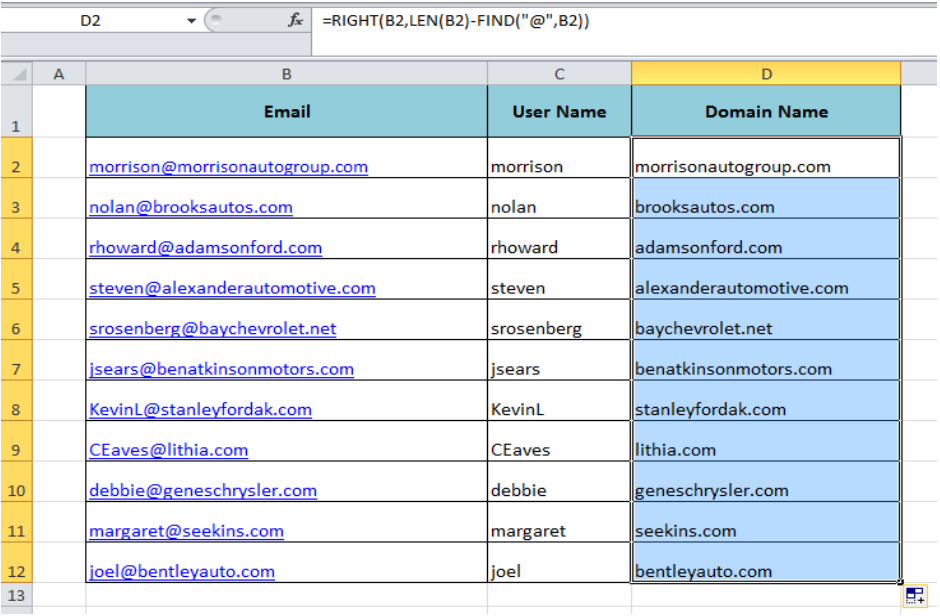Figure 6. Substring Using Right Function

### Using Excel MID Function

The Excel MID function returns the characters from the middle of the string, given a starting position and length. For example, we want to extract the domain name without the .com then we use the MID function along with the FIND function to extract the substring from the middle of the string in the following formula;

`=MID(B2,FIND("@",B2)+1,FIND(".",B2)-FIND("@",B2)-1)  `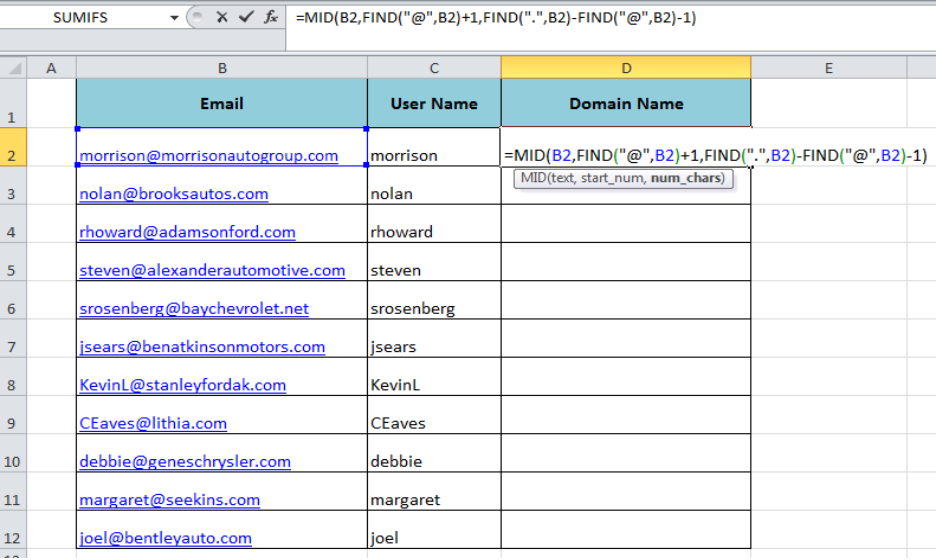Figure 7. Using MID Function

First, we need to specify the starting position of the substring by using the FIND function to return the position of the symbol “@” and add 1. For this we need to supply the following formula in the start_num argument after the text argument of the MID function;

`FIND("@",B2)+1`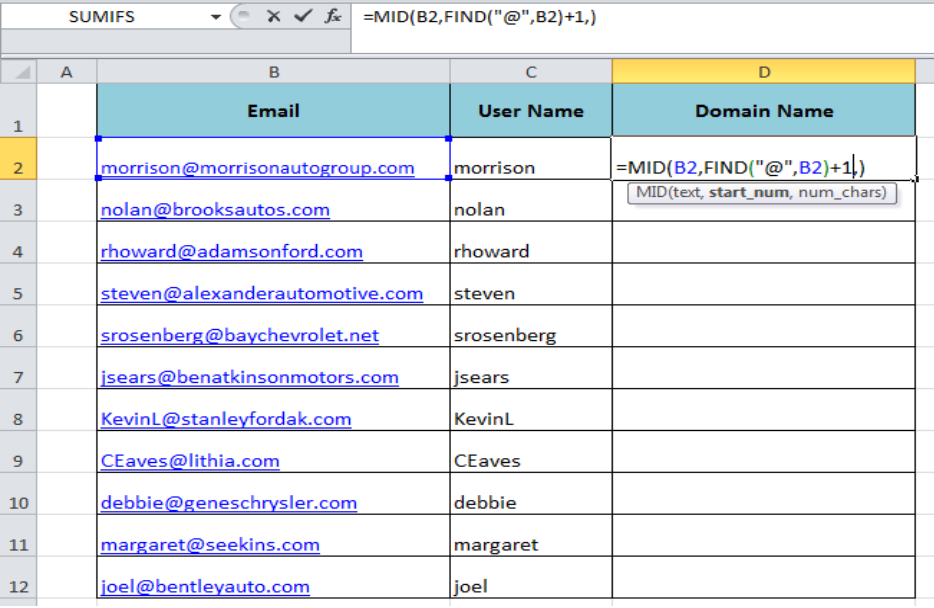Figure 8. Finding the Starting Position of Substring

Then we need to supply the length of the substring to extract from the string. In our example, we again use the FIND function to calculate the length of the substring between symbol “@” and “.” by using the following formula in the num_char argument. This formula returns the number of characters of substring without the .com part.

`FIND(".",B2)-FIND("@",B2)-1`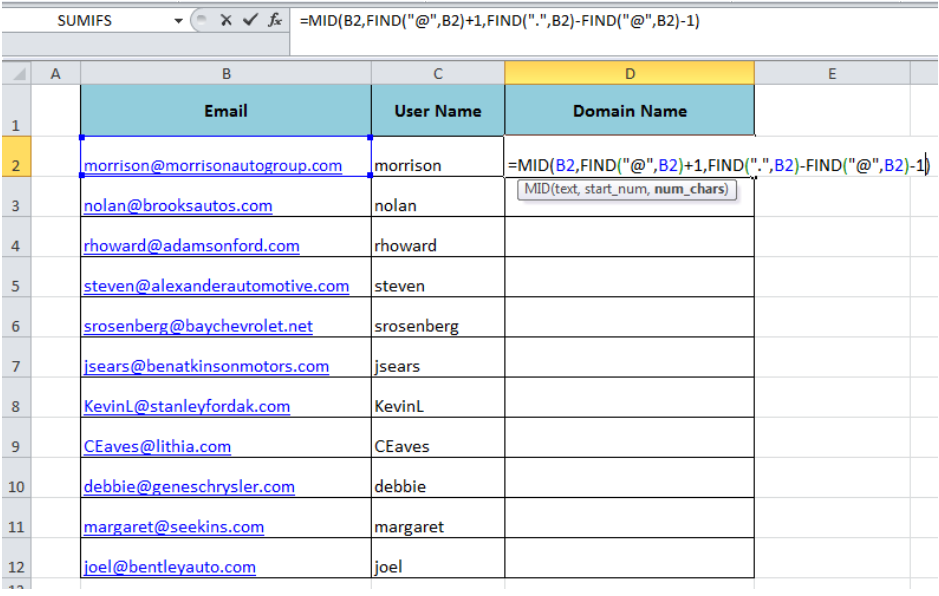Figure 9. Finding Length of Substring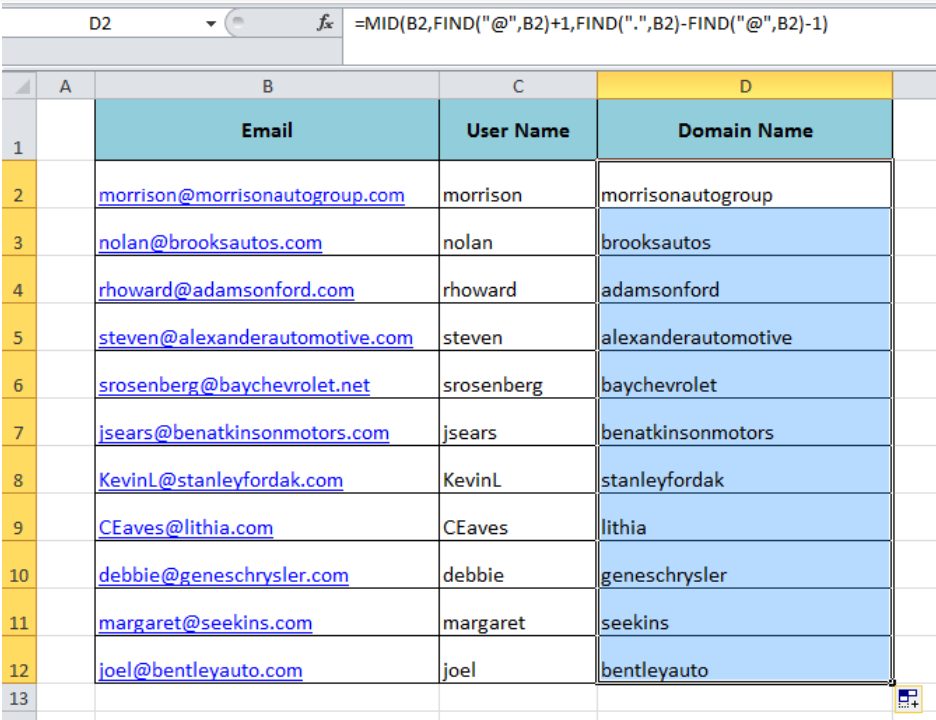Figure 10. Substring MID Function

## Extract Substring Using Excel Text to Column

We can split the string into substring using the specified delimiter characters like “@”, “.” or tab using Text to Column feature in Excel. As we need to split the User Name and Domain Name from email address in our example, therefore we can do it easily by using Text to Column feature in the following steps;

• Select the data and go to Data tab and select Text to Column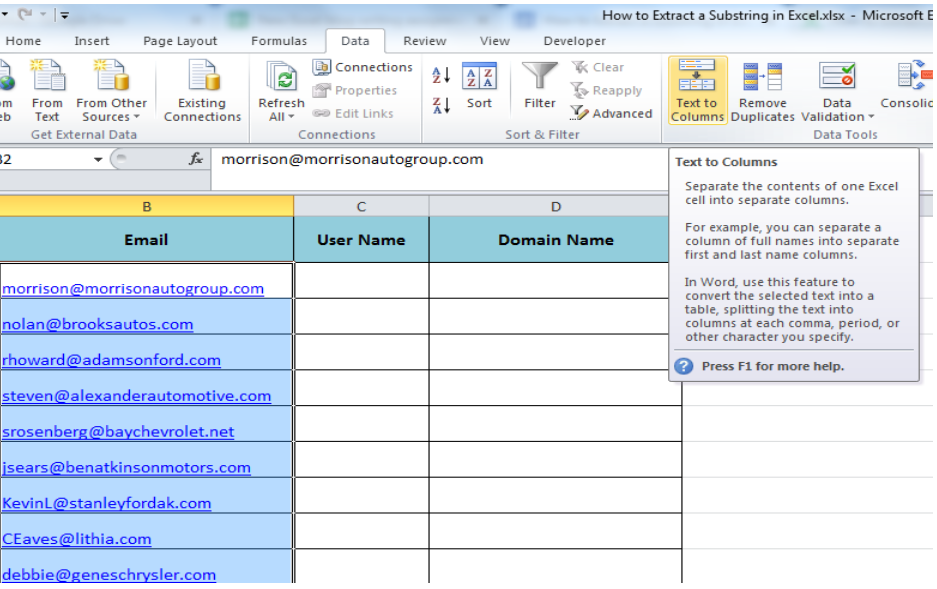Figure 11. Text to Column Feature

• Select Delimited option and press Next button from wizard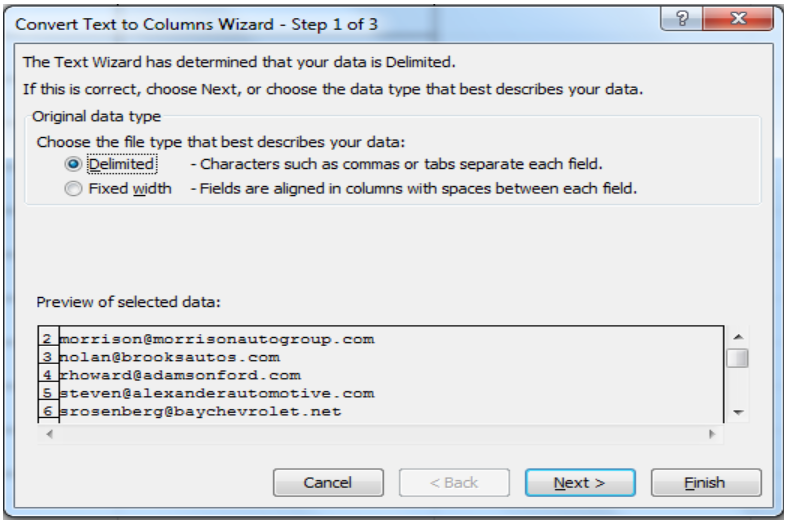Figure 12. Select Delimited Option

• Select Others from delimiters list and enter “@” in the box and press Next button.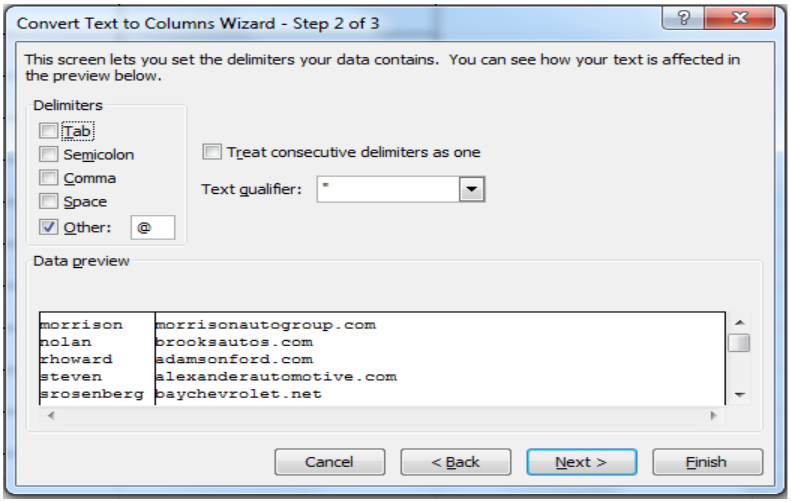Figure 13. Specify the Delimiter

• Select the data format General and select the destination cell where we want to start inserting the substrings, like C2 in our example and press the Finish button.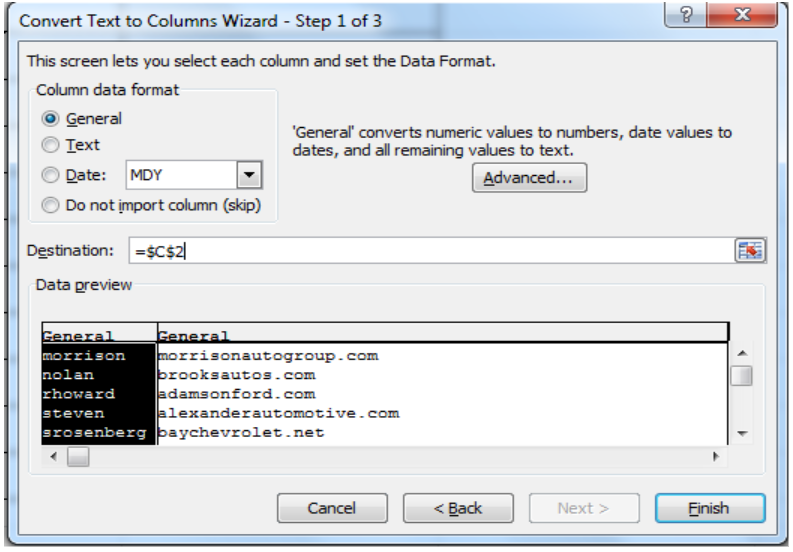Figure 14. Select Destination Cell of Substring

The Excel Text to Column feature splits the string of email address into User Name and Domain Name substrings at the given delimiter of “@” symbol quickly.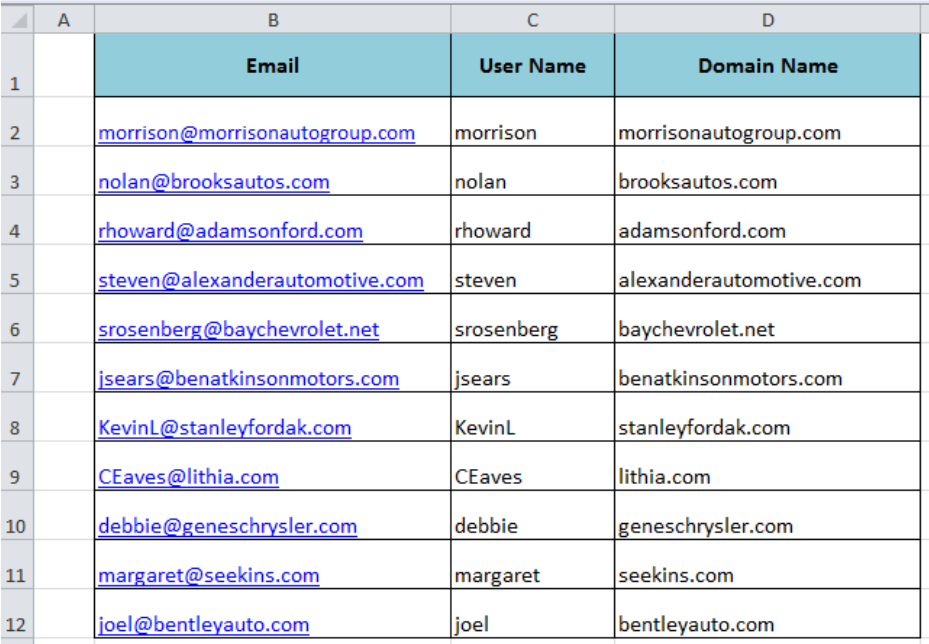Figure 15. Substring

## Instant Connection to an Expert through our Excelchat Service

Most of the time, the problem you will need to solve will be more complex than a simple application of a formula or function. If you want to save hours of research and frustration, try our live Excelchat service! Our Excel Experts are available 24/7 to answer any Excel question you may have. We guarantee a connection within 30 seconds and a customized solution within 20 minutes.